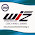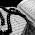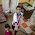## Monday, August 31, 2009

Hi all,

Let's continue our studies in data mining algorithms. In this article, we will see how to use the linear regression to predict values of data series with a simple implementation in Python programming language.

Linear regression is more connected to statistics than computing, it can be used to fit a predictive model to an observed data set of y and x values. After developing such a model, if an additional value of X is then given without its accompanying value of y, the fitted model can be used to make a prediction of the value of y. This model can be shown as a line which best represents the data set. Generally, the problems that linear regression may help are related to prediction of quantity of items at certain moment.

To better understand how the linear regression works, let's see an example. Consider the table 01, which shows the year evolution of the unit price of a product and also the quantity of units sold of this product.

Table 01. History of the unit cost and quantity of sells of a product.

Based on the data set shown at the Table 01, the goal is predict the quantity of products sold when the price (unit cost) of the product achieve the value 2,0. This prediction must consider only the data provided at the table, without considering other possible factors. It's important to notice that all the data provided are fictional, used here only to illustrate the use of the linear regression to solve prediction problems.

To answer this question, we can apply the linear regression. However, nothing guarantees that the Linear Regression will make a "good"prediction, that is, the linear model will fit well to the data. To better understand how it works, let's plot the data shown at the Table 01 into a scatter plot. The Figure 01 shows this graph where the values of the column Unit Cost are placed at the axis X (horizontal) and the values of the quantity sold place at axis y (vertical).Fig. 01. Plot with the unit price x Quantity of products sold (1990-2004)

Analyzing visually the data plot of the Figure 01, we can mentally trace a line that adjust itself to the points. The linear regression just does that: it analyzes the data and mount an line equation so we can predict the next points. One important question to be discussed is: How good it must be this line ? Not only a line that can be generated from analysis of the data. We can imagine a curve (maybe generated by exponential equation) that also adapts itself to the data. In the cases where not ever a curve fit well to the data, we must use a non-linear model. To verify if the data fit well to the linear model, you must use the test called Rˆ2 that checks numerically if it worths or not to use the linear model to the data subset.

There are many ways to use the linear regression. The usual way is use the Microsoft Excel software, which allows to add a line at the plot based on the data points created, as also its correspondent line equation. In this article, we will use a python script implementation of the linear regression algorithm. For further information about the calculation behind of the linear regression, i recommend to the readers a visit to the Kardi Teknomo's website at the link:

First let's suppose that we have the table 01 stored at a simple text file named 'dataset.txt' . Just remembering as we talked earlier, our goal is to obtain the prediction of the quantity of units sold when the price achieve the value of \$\$ 2,00 per unit.

To evaluate this prediction, we use the python script 'linear_reg.py' and at the terminal type the following commands:

>>> python linear_reg.py 'C:/dataset.txt' 'quant_sold' 2.0

The result of the execution of the program is shown at the Figure 02.

>>>y = ax + b (y = 82.9842x + 23.5645)

>>>x = 2 y = 189.533

>>>RR: 0.912826

>>>Linear Model

Figure 02. Result of the execution of the linear regression

It can be noticed that the result of the script printed at the console three lines. The first one showing the line equation, the second the value predicted for the quantity of items sold when the unit cost is \$\$ 2,00 and finally, the third line brings the evaluated Rˆ2 metrics. The fourth line shows the interpretation of the Rˆ2 metrics: if this value is below than 0.8, it's recommended to use a non-linear model. Otherwise, the linear regression can be used to this prediction problem.

One detail that must be considered is the numerical precision of the python implementation, that can be different from the equation presented by the Excel. Other important observation is that we cannot forget that this generated equation not necessarily provide all the scatters of the plot, that is, by using the equation we can not obtain exactly the same values of the previously data, thus the equation generated by the linear regression creates an approximation of the values. The Figure 03 shows the plot with the new value predicted, which it's represented by the red dot.Figure 03. The Quantity of products sold predicted when the price = \$2,00

In this example, we considered that the quantity sold depends only of the unit cost of the product. Based on this supposition, we worked with a price of \$\$ 2,00 for each unit and calculated the quantity approximated by the sells model.

To download the script with all the archives used at this example, just click here.

Any doubts, please comment !

See you next time,

Marcel P. Caraciolo

References:

How to do a Simple Linear Regression with Python

Wikipedia

1.WIZTECH Automation, Anna Nagar, Chennai, has earned reputation offering the best automation training in Chennai in the field of industrial automation. Flexible timings, hands-on-experience, 100% practical. The candidates are given enhanced job oriented practical training in all major brands of PLCs (AB, Keyence, ABB, GE-FANUC, OMRON, DELTA, SIEMENS, MITSUBISHI, SCHNEIDER, and MESSUNG)

PLC training in chennai
Automation training in chennai
Best plc training in chennai
PLC SCADA training in chennai
Process automation training in chennai
Final year eee projects in chennai
VLSI training in chennai

2.Embedded system training: Wiztech Automation Provides Excellent training in embedded system training in Chennai - IEEE Projects - Mechanical projects in Chennai Wiztech provide 100% practical training, Individual focus, Free Accommodation, Placement for top companies. The study also includes standard microcontrollers such as Intel 8051, PIC, AVR, ARM, ARMCotex, Arduino etc.

Embedded system training in chennai
Embedded Course training in chennai
Matlab training in chennai
Android training in chennai
LabVIEW training in chennai
Arduino training in chennai
Robotics training in chennai
Oracle training in chennai
Final year projects in chennai
Mechanical projects in chennai
ece projects in chennai

3.4.5.6.7.8.9.10.Gaining Python certifications will validate your skills and advance your career.
python certification

11.